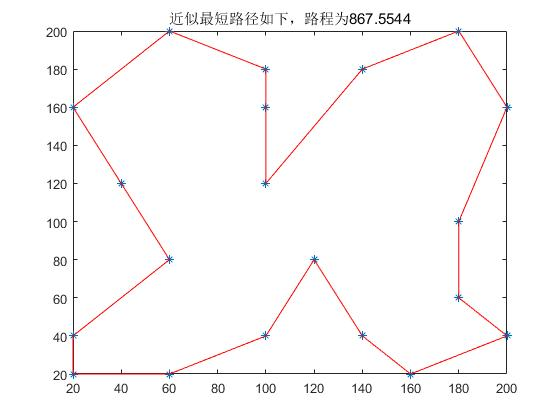## 简介

——《数学建模算法与应用》（司守奎）

## 模型及算法

1. 编码策略

采用十进制编码，用随机数列$w_1，w_2，···，w_{21}$作为染色体，其中$0 \le w_i \le 1(i=2,3,···,20)，w_1=0，w_21=1$；每一个随机序列都和种群中的一个个体一一相对应

2. 初始种群

先利用经典的近似算法——改良圈算法求得一个较好的初始种群。

对于随机产生的初始圈
$$C=C_1···C_{u-1}C_uC_{u+1}···C_{v-1}C_vC_{v+1}···C_{21} ,2 \le u < v \le 20,2 \le C_u <C_v \le 20$$
交换 uv 之间顺序，新的路径为
$$C_1···C_{u-1}C_vC_{v+1}···C_{u+1}C_uC_{v+1}···C_{21}$$
记 $\Delta f=(d_{c_{u-1}c_v}+d_{c_{u}c_{v+1}})-(d_{c_{u-1}c_u}+d_{c_{v}c_{v+1}})$，若$\Delta f<0$，则以新路径修改旧的路径，直到不能够修改为止，就得到一个可行解

直到产生M个可行解，并把这M个可行解转换成染色体编码

3. 目标函数

目标函数为侦察所有目标的路径长度，适应度函数就取为目标函数。要求
$$min f(C_1,···,C_{21})=\sum_{i=1}^{21}d_{c_ic_{i+1}}$$

4. 交叉操作

交叉操作采用单点交叉。对于选定的两个父代个体$f_1=w_1w_2···w_{21}，f_2=w_1^,w_2^,···w_{21}^,$随机的选取第 t 个基因处为交叉点，则经过交叉运算后得到的子代个体为$s_1$和$s_2，s_1$的基因由$f_1$的前 t 个基因和$f_2$的后21 - t个基因构成，$s_2$的基因由$f_2$的前 t 个基因和$f_1$的后21 - t个基因构成。

5. 变异操作

按照给定的变异率，对选定变异的个体，随机的选取三个整数，满足 1<u<v<w<21 ，把 u，v 之间（包括）的基因段插到 w的后面

6. 选择

采用确定性的选择策略，也就是在父代种群和子代种群中选择目标函数值最小的M个个体进化到下一代，这样可以保证父代的优良特性被保存下来。

## Matlab代码